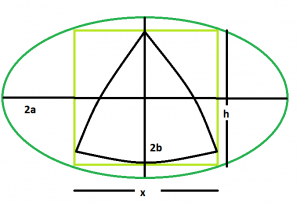GeeksforGeeks App
Open AppBrowser
Continue

# Biggest Reuleaux Triangle inscribed within a square which is inscribed within an ellipse

Given an ellipse with major axis length and minor axis 2a & 2b respectively which inscribes a square which in turn inscribes a reuleaux triangle. The task is to find the maximum possible area of this reuleaux triangle.
Examples:

```Input: a = 5, b = 4
Output: 0.0722389

Input: a = 7, b = 11
Output: 0.0202076```Approach: As, the side of the square inscribed within an ellipse is, x = √(a^2 + b^2)/ab. Please refer Area of the Largest square that can be inscribed in an ellipse.
Also, in the reuleaux triangle, h = x = √(a^2 + b^2)/ab
So, Area of the reuleaux triangle, A = 0.70477*h^2 = 0.70477*((a^2 + b^2)/a^2b^2).
Below is the implementation of the above approach:

## C++

 `// C++ Program to find the biggest Reuleaux triangle``// inscribed within in a square which in turn``// is inscribed within an ellipse``#include ``using` `namespace` `std;` `// Function to find the biggest reuleaux triangle``float` `Area(``float` `a, ``float` `b)``{` `    ``// length of the axes cannot be negative``    ``if` `(a < 0 && b < 0)``        ``return` `-1;` `    ``// height of the reuleaux triangle``    ``float` `h = ``sqrt``(((``pow``(a, 2) + ``pow``(b, 2))``                    ``/ (``pow``(a, 2) * ``pow``(b, 2))));` `    ``// area of the reuleaux triangle``    ``float` `A = 0.70477 * ``pow``(h, 2);` `    ``return` `A;``}` `// Driver code``int` `main()``{``    ``float` `a = 5, b = 4;``    ``cout << Area(a, b) << endl;` `    ``return` `0;``}`

## Java

 `// Java Program to find the biggest Reuleaux triangle``// inscribed within in a square which in turn``// is inscribed within an ellipse``import` `java.io.*;` `class` `GFG``{``    ` `// Function to find the biggest reuleaux triangle``static` `float` `Area(``float` `a, ``float` `b)``{` `    ``// length of the axes cannot be negative``    ``if` `(a < ``0` `&& b < ``0``)``        ``return` `-``1``;` `    ``// height of the reuleaux triangle``    ``float` `h = (``float``)Math.sqrt(((Math.pow(a, ``2``) + Math.pow(b, ``2``))``                ``/ (Math.pow(a, ``2``) * Math.pow(b, ``2``))));` `    ``// area of the reuleaux triangle``    ``float` `A = (``float``)(``0.70477` `* Math.pow(h, ``2``));` `    ``return` `A;``}` `// Driver code``public` `static` `void` `main (String[] args)``{``    ``float` `a = ``5``, b = ``4``;``    ``System.out.println(Area(a, b));``}``}` `// This code is contributed by anuj_67..`

## Python3

 `# Python3 Program to find the biggest Reuleaux``# triangle inscribed within in a square``# which in turn is inscribed within an ellipse``import` `math;` `# Function to find the biggest``# reuleaux triangle``def` `Area(a, b):` `    ``# length of the axes cannot``    ``# be negative``    ``if` `(a < ``0` `and` `b < ``0``):``        ``return` `-``1``;` `    ``# height of the reuleaux triangle``    ``h ``=` `math.sqrt(((``pow``(a, ``2``) ``+` `pow``(b, ``2``)) ``/``                   ``(``pow``(a, ``2``) ``*` `pow``(b, ``2``))));` `    ``# area of the reuleaux triangle``    ``A ``=` `0.70477` `*` `pow``(h, ``2``);` `    ``return` `A;` `# Driver code``a ``=` `5``;``b ``=` `4``;``print``(``round``(Area(a, b), ``7``));` `# This code is contributed by chandan_jnu`

## C#

 `// C# Program to find the biggest Reuleaux triangle``// inscribed within in a square which in turn``// is inscribed within an ellipse``using` `System;` `class` `GFG``{``    ` `// Function to find the biggest reuleaux triangle``static` `double` `Area(``double` `a, ``double` `b)``{` `    ``// length of the axes cannot be negative``    ``if` `(a < 0 && b < 0)``        ``return` `-1;` `    ``// height of the reuleaux triangle``    ``double` `h = (``double``)Math.Sqrt(((Math.Pow(a, 2) +``                                    ``Math.Pow(b, 2)) /``                                   ``(Math.Pow(a, 2) *``                                   ``Math.Pow(b, 2))));` `    ``// area of the reuleaux triangle``    ``double` `A = (``double``)(0.70477 * Math.Pow(h, 2));` `    ``return` `A;``}` `// Driver code``static` `void` `Main()``{``    ``double` `a = 5, b = 4;``    ``Console.WriteLine(Math.Round(Area(a, b),7));``}``}` `// This code is contributed by chandan_jnu`

## PHP

 ``

## Javascript

 ``

Output:

`0.0722389`

Time Complexity: O(logn) as it is using inbuilt sqrt function

Auxiliary Space: O(1)

My Personal Notes arrow_drop_up